• 一、问题上篇文章中我们已经搞明白了逻辑回归的问题中，xgboost的train（）,fit（）方法已经不同参数的objective的对应的输出结果和关系，这篇文章我们就来研究下xgboost再做多分类时，train(),fit（）建模后输出的...

一、问题

上篇文章中我们已经搞明白了逻辑回归的问题中，xgboost的train（）,fit（）方法以及不同参数的objective的对应的输出结果和关系，这篇文章我们就来研究下xgboost再做多分类时，train(),fit（）建模后输出的值是怎么样的，它都有那些objective的参数，并且分别对应着怎样的输出？

二、objective参数比较

xgboost的官方文档关于多分类的objective如下：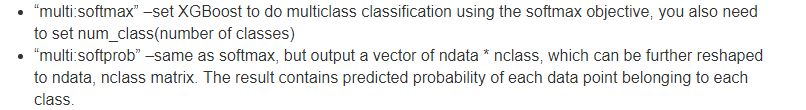从文档中我们可以看出，multi：softmax是使用softmax后产生的分类结果，而multi:softprob是输出的概率矩阵。我们这里以标签为0,1,2的三分类数据为例，前者输出的结果是一维数据，0,1,2的分类标签；后者输出的是n*3的概率矩阵（n为测试数据的条数）。

这两个参数之间有什么关系呢？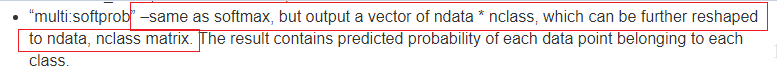从这里我们可以看出，最终的分类标签是从概率矩阵中得到的，在n*3的概率矩阵中（如下图）：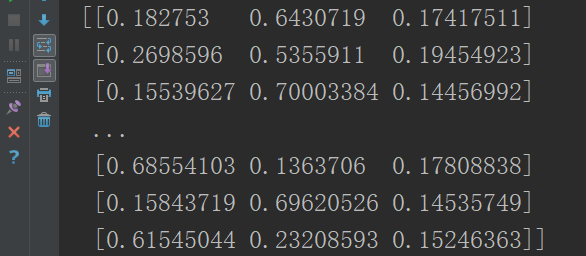我们可以看出概率矩阵中每行数据的数值相加得1，我们取每行数据中概率值最大数值的下标作为最终的分类标签，然后给multi:softmax的输出结果做对比看是否一致。

xgb_params = {
'learning_rate': 0.1,  # 步长
'n_estimators': 10,
'max_depth': 5,  # 树的最大深度
'objective': 'multi:softprob',
'num_class': 3,
'min_child_weight': 1,  # 决定最小叶子节点样本权重和，如果一个叶子节点的样本权重和小于min_child_weight则拆分过程结束。
'gamma': 0,  # 指定了节点分裂所需的最小损失函数下降值。这个参数的值越大，算法越保守
'silent': 0,  # 输出运行信息
'subsample': 0.8,  # 每个决策树所用的子样本占总样本的比例（作用于样本）
'colsample_bytree': 0.8,  # 建立树时对特征随机采样的比例（作用于特征）典型值：0.5-1
'seed': 27}
print "training..."
model = xgb.train(xgb_params, xgb.DMatrix(x_train, y_train))
softprob_pred = model.predict(xgb.DMatrix(x_test))
print softprob_pred
# 处理概率矩阵-得到最终的预测标签
indexlist = []
for item in softprob_pred:
maxnum = max(item)
item = np.where(item == maxnum)
indexlist.append(item.item())
################################
xgb_params = {
'learning_rate': 0.1,  # 步长
'n_estimators': 20,
'max_depth': 5,  # 树的最大深度
'objective': 'multi:softmax',
'num_class': 3,
'min_child_weight': 1,  # 决定最小叶子节点样本权重和，如果一个叶子节点的样本权重和小于min_child_weight则拆分过程结束。
'gamma': 0,  # 指定了节点分裂所需的最小损失函数下降值。这个参数的值越大，算法越保守
'silent': 0,  # 输出运行信息
'subsample': 0.8,  # 每个决策树所用的子样本占总样本的比例（作用于样本）
'colsample_bytree': 0.8,  # 建立树时对特征随机采样的比例（作用于特征）典型值：0.5-1
'seed': 27}
print "training..."
model = xgb.train(xgb_params, xgb.DMatrix(x_train, y_train))
softmax_pred = model.predict(xgb.DMatrix(x_test))
count = 0
for i in np.arange(0, len(softmax_pred)):
if (softmax_pred[i] == indexlist[i]):
count += 1
print "len:", len(softmax_pred)
print "count:", count
if count == len(softmax_pred):
print "true"
else:
print "false"

输出的结果：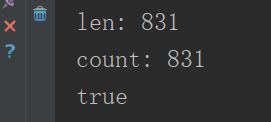由此可见，最终的预测标签就是通过取概率矩阵的每行数据最大值的下标获得的。

那么问题来了，我们知道像xgboost这类集成树算法，他本身用到的是回归树，这就决定了它本身就是用来做回归的，调整后可以用来做分类，对于多分类来说是通过softmax函数把回归结果映射成最终的多分类标签的。我们把objective改成线性回归的参数（reg:linear），看下结果如何？如何通过softmax函数转换成最终分类标签？

输出结果如下：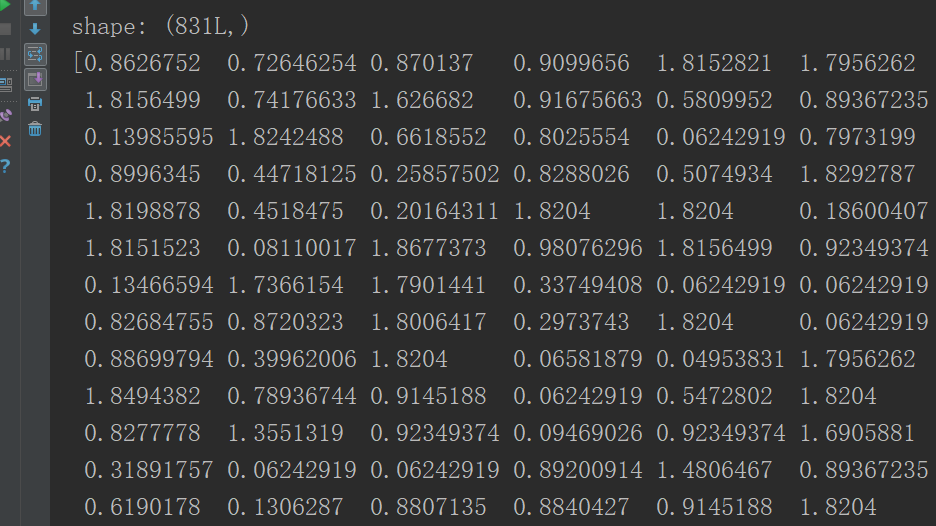得到的是一个一维0-2之间的预测值，怎么映射成一个831*3的矩阵呢？

对于softmax函数它的输入和输出是相同的shape，对于一个831*1的数据，得到的也是一个值都在0-1之间的831*1的数据，根本无法直接这样得到概率矩阵。这个问题有想法的同学可以加QQ群:821675528交流！

三、多分类问题中fit()和train()的结果

用相同的数据，相同的参数（objective:multi:softmax），分别用fit()和train（）方法得到的输出结果是一致的，都是预测的0,1,2的标签，实验过程如下：

clf = XGBClassifier(
n_estimators=20,  # 迭代次数
learning_rate=0.1,  # 步长
max_depth=5,  # 树的最大深度
min_child_weight=1,  # 决定最小叶子节点样本权重和
silent=1,  # 输出运行信息
subsample=0.8,  # 每个决策树所用的子样本占总样本的比例（作用于样本）
colsample_bytree=0.8,  # 建立树时对特征随机采样的比例（作用于特征）典型值：0.5-1
objective='multi:softmax',  # 多分类！！！！！！
num_class=3,
seed=27)
print "training..."
clf.fit(x_train, y_train, verbose=True)
fit_pred = clf.predict(x_test)
print fit_pred
xgb_params = {
'learning_rate': 0.1,  # 步长
'max_depth': 5,  # 树的最大深度
'objective': 'multi:softmax',
'num_class': 3,
'min_child_weight': 1,  # 决定最小叶子节点样本权重和，如果一个叶子节点的样本权重和小于min_child_weight则拆分过程结束。
'silent': 0,  # 输出运行信息
'subsample': 0.8,
'colsample_bytree': 0.8,  # 建立树时对特征随机采样的比例（作用于特征）典型值：0.5-1
'seed': 27}
print "training..."
model = xgb.train(xgb_params, xgb.DMatrix(x_train, y_train), num_boost_round=20)
train_pred = model.predict(xgb.DMatrix(x_test))
count = 0
print train_pred
# 判断train_pred和fit_pred是否一致
for i in np.arange(0, len(train_pred)):
if (train_pred[i] == fit_pred[i]):
count += 1
print "len:", len(train_pred)
print "count:", count
if count == len(train_pred):
print "true"
else:
print "false"

实验结果如下：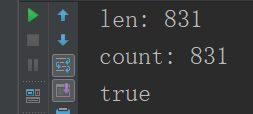总结：综上我们可以看出xgboost多分类问题就是通过softmax函数做映射得到最终的分类标签，只是怎么把回归的结果映射成概率矩阵，我还没有搞清楚，有好的想法或者同样疑问的同学可以加QQ群：821675528交流！！

展开全文phyllisyuell 2018-07-12 19:12:49
• xgboost objective和eval_metric的区别 机器学习 xgboost

之前对于xgboost中的两个参数objective和eval_metric一直分不清楚，不知道它们各自的作用。后来通过查找资料理清了他们之间的关系。这里记录一下。 1、objective objective参数代表的是模型的损失函数，也就是我们...

之前对于xgboost中的两个参数objective和eval_metric一直分不清楚，不知道它们各自的作用。后来通过查找资料理清了他们之间的关系。这里记录一下。

1、objective

objective参数代表的是模型的损失函数，也就是我们进行优化的目标。

这里要注意损失函数与目标函数的区别，一般情况下，我们说的损失函数就是目标函数，但是一些模型如xgboost要求目标函数要有一阶导数和二阶导数才能进行优化，而很多目标函数是没有一阶导数或二阶导数的，所以这时就用具有相似结果的损失函数来代替（如果损失函数能够很好地替代目标函数，这是最好的情况，如果替代效果不佳，则影响模型优化）。在模型优化中，我们使用的都是损失函数。
objective是训练模型必备的函数，只有定义了objective模型才能进行训练

2、eval_metric函数
eval_metric是评价函数，对模型的训练没有影响，而是在模型训练完成之后评估模型效果。如我们经常使用logloss作为objective，经常与之搭配的评价函数是auc、acc等。

为什么评价的时候不用objective呢？本来就是用objective来优化的，自己评价自己就没多大意义了，所以一般需要来自另一个评分机制的eval_metric来评估模型效果。

eval_metric对于训练模型没有影响，即使我们不定义这个函数模型也可以正常训练。

展开全文YangTinTin 2020-06-28 13:52:50
• 熟悉xgboost的小伙伴都知道，它在训练模型时，有train（）方法和fit（）方法，train方法使用如下：params ={'eta': 0.1, 'max_depth': 4, 'num_boost_round':20, 'objective': 'reg:logistic', 'random_state': ...

一、问题

熟悉xgboost的小伙伴都知道，它在训练模型时，有train（）方法和fit（）方法，两种方法都是用于构建模型的，然而在使用过程中有什么不同的地方呢，这篇文章带领大家一起来看一下。train方法使用如下：

params ={'eta': 0.1,
'max_depth': 4,
'num_boost_round':20,
'objective': 'reg:logistic',
'random_state': 27,
'silent':0
}
model = xgb.train(params,xgb.DMatrix(x_train, y_train))
train_pred=model.predict(xgb.DMatrix(x_test))

而fit方法是直接使用xgboost封装好的XGBClassifier或者XGBRegressor时使用：

model = XGBClassifier(
learning_rate=0.1,
n_estimators=20,
max_depth=4,
objective='binary:logistic',
seed=27,
silent=0
)
model.fit(x_train,y_train,verbose=True)
fit_pred=model.predict(x_test)
print fit_pred

相同的数据集，相同的参数得到的预测值却是不一样的，fit_pred的值是0,1的具体的预测标签，train_pred的值是0-1之间的概率值；为什么结果是不一样的呢？如何把0-1之间的概率值映射成0,1标签呢？这个后面揭晓，我们先看下，xgboost中用于做逻辑回归的objective的参数都有哪些，得到预测结果有什么不同！

二、objective参数比较

xgboost官方文档关于逻辑回归objective有三个参数，如下：1、reg:logistic PK binary:logistic

实际上reg:logistic，binary:logistic都是输出逻辑回归的概率值，实验过程如下：

params ={'eta': 0.1,
'max_depth': 4,
'num_boost_round':20,
'objective': 'binary:logistic',
'random_state': 27,
'silent':0
}
model = xgb.train(params,xgb.DMatrix(x_train, y_train))
bin_log_pred=model.predict(xgb.DMatrix(x_test))
# print bin_log_pred
###################################
params ={'eta': 0.1,
'max_depth': 4,
'num_boost_round':20,
'objective': 'reg:logistic',
'random_state': 27,
'silent':0
}
model = xgb.train(params,xgb.DMatrix(x_train, y_train))
reg_log_pred=model.predict(xgb.DMatrix(x_test))
# print reg_log_pred
count=0
for i in np.arange(0,len(reg_log_pred)):
if (reg_log_pred[i]==bin_log_pred[i]):
count+=1
print "len:",len(reg_log_pred)
print "count:",count
if count==len(reg_log_pred):
print "true"
else:
print "false"

输出结果：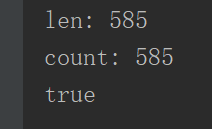2、reg:logistic  PK  binary:logitraw

然后我们再来看下binary:logitraw参数，由文档可以看出这个参数得到的是集成树模型最后输出的得分，即转换成概率值之前的值，我们知道xgboost这类集成树算法用到的回归树，这就决定了它本身就是用来做回归的，调整后可以用来做分类，这里的调整是指通过sigmoid函数处理后来做二分类，通过softmax函数处理后来做多分类。于是，我们定义一个sigmoid函数，把binary:logitraw参数输出的得分通过sigmoid函数映射成概率值，对比下是否和reg:logistic参数得到的概率值是一样的。

params ={'eta': 0.1,
'max_depth': 4,
'num_boost_round':20,
'objective': 'reg:logistic',
'random_state': 27,
'silent':0
}
model = xgb.train(params,xgb.DMatrix(x_train, y_train))
reg_log_pred=model.predict(xgb.DMatrix(x_test))
# print reg_log_pred
###################################
params ={'eta': 0.1,
'max_depth': 4,
'num_boost_round':20,
'objective': 'binary:logitraw',
# 'n_jobs': 4,
'random_state': 27,
'silent':0
}
model = xgb.train(params,xgb.DMatrix(x_train, y_train))
logitraw_pred=model.predict(xgb.DMatrix(x_test))
print logitraw_pred
def sigmoid(x):
return 1./(1.+np.exp(-x))
y=sigmoid(logitraw_pred)
print y
count=0
for i in np.arange(0,len(reg_log_pred)):
if (reg_log_pred[i]==y[i]):
count+=1
print len(reg_log_pred)
print count
if count==len(reg_log_pred):
print "true"
else:
print "false"

输出结果：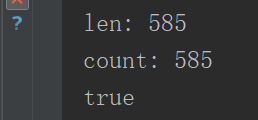3、总结

上述实验，通过使用相同的数据集，相同的参数（除了objective参数，其他相同），研究了逻辑回归的objective的三种不同参数的输出结果，得到如下结论：

1、binary:logistic和 'objective': 'reg:logistic'的输出是一样的,都是预测的概率

2、binary:logitraw是输出的得分，用sigmoid（）函数处理后就和上述两个概率值一致

三、XGBClassifier都做了些什么

回到我们一开始的问题，xgboost train()方法得到概率值之后，如何处理成最终的0,1标签呢？

这个工作应该是在XGBClassifier中完成的，我们进行下列实验进行验证：

model = XGBClassifier(
learning_rate=0.1,
n_estimators=20,
max_depth=4,
objective='binary:logistic',
seed=27,
silent=0
)
model.fit(x_train,y_train,verbose=True)
fit_pred=model.predict(x_test)
print fit_pred
#######################
params ={'learning_rate': 0.1,
'max_depth': 4,
'objective': 'reg:logistic',
'random_state': 27,
'silent':0
}
model = xgb.train(params,xgb.DMatrix(x_train, y_train),num_boost_round=20)
train_pred=model.predict(xgb.DMatrix(x_test))
#概率值的阈值假设为0.5，小于等于0.5的预测为0，否则预测为1
for i in np.arange(0,len(train_pred)):
if train_pred[i]<0.5:
train_pred[i]=0
else:
train_pred[i]=1
print train_pred
print type(train_pred)
count=0
for i in np.arange(0,len(train_pred)):
if (train_pred[i]==fit_pred[i]):
count+=1
print len(train_pred)
print count
if count==len(train_pred):
print "true"
else:
print "false"

输出的结果：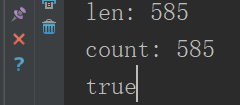由此可见我们的假设是成立的，XGBClassifier里就是把预测的概率值，取阈值0.5，小于这个值的为0，大于这个值的为1，实验过程中还发现并没有等于0.5的概率值，我的设想是对于一个二分类问题，把概率预测成0.5没有任何意义o(*￣︶￣*)o

为了增加可信度，我们把训练数据放到模型里进行预测，并对于，train和fit输出的预测结果，结果如下：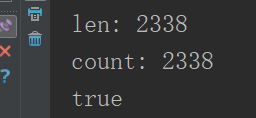通过上述实验，我们对xgboost算法做逻辑回归问题应该有了更加深入的认识，下篇文章我们一起来研究下xgboost在处理多分类问题的过程。

（发现文章中有任何问题请联系：phyllisyuell@163.com）

展开全文phyllisyuell 2018-07-11 22:26:53

Objective

自定义Objective函数示例：链接

# user define objective function, given prediction, return gradient and second order gradient
# this is loglikelihood loss
logregobj < -  function（preds，dtrain）{
labels< -  getinfo（dtrain，“label”）
preds < -  1 /1 + exp（-preds））
grad < -  preds  - labels
hess < -  preds *1  -  preds）
}

Objective是训练的关键函数，任何xgboost模型都需要定义它才能训练。Objective函数直接用于每个树中的每个节点处的拆分过程。

feval

xgboost中的feval在优化或训练模型方面没有任何的直接作用。你甚至不需要它就能训练。它不会影响分裂。它所做的只是在训练结束后对你的模型进行评分。下面是自定义feval的例子

evalerror < -  function（preds，dtrain）{
labels< -  getinfo（dtrain，“label”）
err < -  as.numeric（sum（labels！=（preds> 0）））/ length（labels）
returnlist（metric =“error”，value = err））  #译注：metric为名称，value为分数
}

请注意，它只返回一个名称（metric）和一个分数（value）。通常情况下，fevalobjective可能是相同的。但是有时候，你可能需要有点不同的评分机制，或者没有导数。例如，人们使用logloss作为Objective进行训练，但使用AUC 作为feval来评估模型。

另外，你可以使用feval在模型已经没有改进的时候停止训练。你还可以使用多个feval函数以不同的方式为你的模型打分并观察它们。

总的来说，你不需要feval函数来训练模型，而只是为了评估它，并帮助它尽早停止训练。

展开全文xpy870663266 2019-05-22 11:48:07
• 从L2R开始理解一下xgboost的 'objective': 'rank:pairwise'参数 xgboost

weixin_42001089 2018-11-17 12:48:57
• xgboost建模输入objective='reg:linear'输出变'multi:softprob'，是哪里出问题了？ 机器学习 python

weixin_46457241 2020-10-13 16:30:21
• qq_42363032 2021-05-17 15:48:53
• 解决xgboost.core.XGBoostError: [17:12:src/objective/objective.cc:23: Unknown objective function ... 机器学习 debug

weixin_40539826 2020-12-26 20:52:30
• weixin_35710893 2021-01-14 00:49:53
• XGBOOST从原理到实战：二分类 、多分类 xgboost 二分类 多分类 python 算法

HHTNAN 2018-07-17 12:49:05
• leokingszx 2017-12-26 22:05:29
• 33KB weixin_38626473 2021-01-20 11:54:20
• weixin_44261347 2020-11-01 21:40:39
• weixin_36164428 2021-03-05 13:57:46
• weixin_39661353 2020-12-18 23:25:20
• weixin_42575593 2019-02-01 14:13:14
• weixin_41358871 2018-08-09 17:53:43
• xunileida 2017-09-19 18:06:34
• weixin_39945795 2020-12-18 23:25:17
• weixin_39830012 2020-12-23 14:38:10
• Xgboost参数介绍 算法 人工智能 Xgboost

csdn_elsa 2020-12-07 11:16:24
• 机器学习：xgboost原理及实践 xgboost

qq_29317617 2019-04-27 21:38:00
• weixin_29479879 2021-01-28 19:40:37
• XGBoost python调参示例 python XGBoost 调参

weiyongle1996 2017-10-27 09:11:38
• weixin_39716105 2020-12-09 21:11:49...